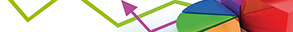Journal metrics
Acceptance rate7%
Submission to final decision36 days
Acceptance to publication33 days
CiteScore0.560
Impact Factor-

### Generalized Autoregressive Conditional Heteroskedastic Model to Examine Silver Price Volatility and Its Macroeconomic Determinant in Ethiopia Market

####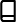Journal profile

Journal of Probability and Statistics publishes papers on the theory and application of probability and statistics that consider new methods and approaches to their implementation, or report significant results for the field.

####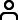Editor spotlight

Journal of Probability and Statistics maintains an Editorial Board of practicing researchers from around the world, to ensure manuscripts are handled by editors who are experts in the field of study.

####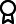Special Issues

We currently have a number of Special Issues open for submission. Special Issues highlight emerging areas of research within a field, or provide a venue for a deeper investigation into an existing research area.

## Latest Articles

More articles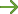Research Article

### Distributions of the Ratio and Product of Two Independent Weibull and Lindley Random Variables

In this paper, we derive the cumulative distribution functions (CDF) and probability density functions (PDF) of the ratio and product of two independent Weibull and Lindley random variables. The moment generating functions (MGF) and the k-moment are driven from the ratio and product cases. In these derivations, we use some special functions, for instance, generalized hypergeometric functions, confluent hypergeometric functions, and the parabolic cylinder functions. Finally, we draw the PDF and CDF in many values of the parameters.

Research Article

### T-Dagum: A Way of Generalizing Dagum Distribution Using Lomax Quantile Function

Recently, different distributions have been generalized using the -R {Y} framework but the possibility of using Dagum distribution has not been assessed. The -R {Y} combines three distributions, with one as a baseline distribution, with the strength of each distribution combined to produce greater effect on the new generated distribution. The new generated distributions would have more parameters but would have high flexibility in handling bimodality in datasets and it is a weighted hazard function of the baseline distribution. This paper therefore generalized the Dagum distribution using the quantile function of Lomax distribution. A member of -Dagum class of distribution called exponentiated-exponential-Dagum {Lomax} (EEDL) distribution was proposed. The distribution will be useful in survival analysis and reliability studies. Different characterizations of the distribution are derived, such as the asymptotes, stochastic ordering, stress-strength analysis, moment, Shannon entropy, and quantile function. Simulated and real data are used and compared favourably with existing distributions in the literature.

Research Article

### Estimation of a Finite Population Mean under Random Nonresponse Using Kernel Weights

Nonresponse is a potential source of errors in sample surveys. It introduces bias and large variance in the estimation of finite population parameters. Regression models have been recognized as one of the techniques of reducing bias and variance due to random nonresponse using auxiliary data. In this study, it is assumed that random nonresponse occurs in the survey variable in the second stage of cluster sampling, assuming full auxiliary information is available throughout. Auxiliary information is used at the estimation stage via a regression model to address the problem of random nonresponse. In particular, auxiliary information is used via an improved Nadaraya–Watson kernel regression technique to compensate for random nonresponse. The asymptotic bias and mean squared error of the estimator proposed are derived. Besides, a simulation study conducted indicates that the proposed estimator has smaller values of the bias and smaller mean squared error values compared to existing estimators of a finite population mean. The proposed estimator is also shown to have tighter confidence interval lengths at coverage rate. The results obtained in this study are useful for instance in choosing efficient estimators of a finite population mean in demographic sample surveys.

Research Article

### Forecasting the Covolatility of Coffee Arabica and Crude Oil Prices: A Multivariate GARCH Approach with High-Frequency Data

Forecasting the covolatility of asset return series is becoming the subject of extensive research among academics, practitioners, and portfolio managers. This paper estimates a variety of multivariate GARCH models using weekly closing price (in USD/barrel) of Brent crude oil and weekly closing prices (in USD/pound) of Coffee Arabica and compares the forecasting performance of these models based on high-frequency intraday data which allows for a more precise realized volatility measurement. The study used weekly price data to explicitly model covolatility and employed high-frequency intraday data to assess model forecasting performance. The analysis points to the conclusion that the varying conditional correlation (VCC) model with Student’s t distributed innovation terms is the most accurate volatility forecasting model in the context of our empirical setting. We recommend and encourage future researchers studying the forecasting performance of MGARCH models to pay particular attention to the measurement of realized volatility and employ high-frequency data whenever feasible.

Research Article

### Stochastic Restricted LASSO-Type Estimator in the Linear Regression Model

Among several variable selection methods, LASSO is the most desirable estimation procedure for handling regularization and variable selection simultaneously in the high-dimensional linear regression models when multicollinearity exists among the predictor variables. Since LASSO is unstable under high multicollinearity, the elastic-net (Enet) estimator has been used to overcome this issue. According to the literature, the estimation of regression parameters can be improved by adding prior information about regression coefficients to the model, which is available in the form of exact or stochastic linear restrictions. In this article, we proposed a stochastic restricted LASSO-type estimator (SRLASSO) by incorporating stochastic linear restrictions. Furthermore, we compared the performance of SRLASSO with LASSO and Enet in root mean square error (RMSE) criterion and mean absolute prediction error (MAPE) criterion based on a Monte Carlo simulation study. Finally, a real-world example was used to demonstrate the performance of SRLASSO.

Research Article

### The Improved Value-at-Risk for Heteroscedastic Processes and Their Coverage Probability

A risk measure commonly used in financial risk management, namely, Value-at-Risk (VaR), is studied. In particular, we find a VaR forecast for heteroscedastic processes such that its (conditional) coverage probability is close to the nominal. To do so, we pay attention to the effect of estimator variability such as asymptotic bias and mean square error. Numerical analysis is carried out to illustrate this calculation for the Autoregressive Conditional Heteroscedastic (ARCH) model, an observable volatility type model. In comparison, we find VaR for the latent volatility model i.e., the Stochastic Volatility Autoregressive (SVAR) model. It is found that the effect of estimator variability is significant to obtain VaR forecast with better coverage. In addition, we may only be able to assess unconditional coverage probability for VaR forecast of the SVAR model. This is due to the fact that the volatility process of the model is unobservable.Journal metrics
Acceptance rate7%
Submission to final decision36 days
Acceptance to publication33 days
CiteScore0.560
Impact Factor-Author guidelinesEditorial boardDatabases and indexing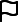Sign up for content alertsSign upAuthor guidelinesEditorial boardDatabases and indexingSign up for content alertsSign up

We are committed to sharing findings related to COVID-19 as quickly and safely as possible. Any author submitting a COVID-19 paper should notify us at help@hindawi.com to ensure their research is fast-tracked and made available on a preprint server as soon as possible. We will be providing unlimited waivers of publication charges for accepted articles related to COVID-19. Sign up here as a reviewer to help fast-track new submissions.